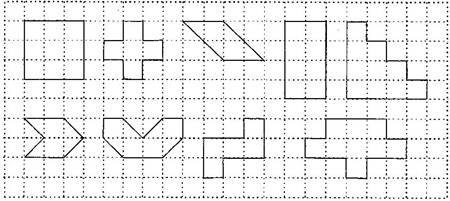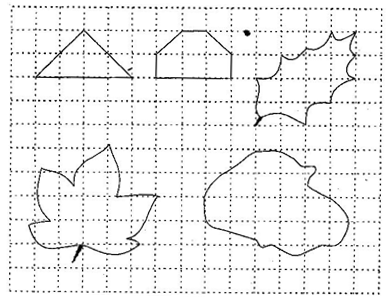1. /
2. CBSE
3. /
4. Class 06
5. /
6. Mathematics
7. /
8. NCERT Solutions for Class...

# NCERT Solutions for Class 6 Maths Exercise 10.2

NCERT solutions for Maths Mensuration## NCERT Solutions for Class 6 Maths Mensuration

###### Question 1.Find the areas of the following figures by counting squares:(a) Number of filled square = 9

{tex}\therefore {/tex} Area covered by squares = 9 x 1 = 9 sq. units

(b) Number of filled squares = 5

{tex}\therefore {/tex} Area covered by filled squares = 5 x 1 = 5 sq. units

(c) Number of full filled squares = 2

Number of half filled squares = 4

{tex}\therefore {/tex} Area covered by full filled squares = 2 x 1 = 2 sq. units

And Area covered by half filled squares = x = 2 sq. units

{tex}\therefore {/tex} Total area = 2 + 2 = 4 sq. units

(d) Number of filled squares = 8

{tex}\therefore {/tex} Area covered by filled squares = 8 x 1 = 8 sq. units

(e) Number of filled squares = 10

{tex}\therefore {/tex} Area covered by filled squares = 10 x 1 = 10 sq. units

(f) Number of full filled squares = 2

Number of half filled squares = 4

{tex}\therefore {/tex} Area covered by full filled squares = 2 x 1 = 2 sq. units

And Area covered by half filled squares = x = 2 sq. units

{tex}\therefore {/tex} Total area = 2 + 2 = 4 sq. units

(g)Number of full filled squares = 4

Number of half filled squares = 4

{tex}\therefore {/tex} Area covered by full filled squares = 4 x 1 = 4 sq. units

And Area covered by half filled squares = x = 2 sq. units

{tex}\therefore {/tex} Total area = 4 + 2 = 6 sq. units

(h)Number of filled squares = 5

{tex}\therefore {/tex} Area covered by filled squares = 5 x 1 = 5 sq. units

(i)Number of filled squares = 9

{tex}\therefore {/tex} Area covered by filled squares = 9 x 1 = 9 sq. units

(j)Number of full filled squares = 2

Number of half filled squares = 4

{tex}\therefore {/tex} Area covered by full filled squares = 2 x 1 = 2 sq. units

And Area covered by half filled squares = x = 2 sq. units

{tex}\therefore {/tex} Total area = 2 + 2 = 4 sq. units

(k)Number of full filled squares = 4

Number of half filled squares = 2

{tex}\therefore {/tex} Area covered by full filled squares = 4 x 1 = 4 sq. units

And Area covered by half filled squares = x = 1 sq. units

{tex}\therefore {/tex} Total area = 4 + 1 = 5 sq. units

(l)Number of full filled squares = 3

Number of half filled squares = 10

{tex}\therefore {/tex} Area covered by full filled squares = 3 x 1 = 3 sq. units

And Area covered by half filled squares = x = 5 sq. units

{tex}\therefore {/tex} Total area = 3 + 5 = 8 sq. units

(m)Number of full filled squares = 7

Number of half filled squares = 14

{tex}\therefore {/tex} Area covered by full filled squares = 7 x 1 = 7 sq. units

And Area covered by half filled squares = x = 7 sq. units

{tex}\therefore {/tex} Total area = 7 + 7 = 14 sq. units

(n)Number of full filled squares = 10

Number of half filled squares = 16

{tex}\therefore {/tex} Area covered by full filled squares = 10 x 1 = 10 sq. units

And Area covered by half filled squares = x = 8 sq. units

{tex}\therefore {/tex} Total area = 10 + 8 = 18 sq. units

## NCERT Solutions for Class 6 Maths Exercise 10.2

NCERT Solutions Class 6 Maths PDF (Download) Free from myCBSEguide app and myCBSEguide website. Ncert solution class 6 Maths includes text book solutions from Class 6 Maths Book . NCERT Solutions for CBSE Class 6 Maths have total 14 chapters. 6 Maths NCERT Solutions in PDF for free Download on our website. Ncert Maths class 6 solutions PDF and Maths ncert class 6 PDF solutions with latest modifications and as per the latest CBSE syllabus are only available in myCBSEguide.

## CBSE app for Students

To download NCERT Solutions for Class 6 Maths, Social Science Computer Science, Home Science, Hindi English, Maths Science do check myCBSEguide app or website. myCBSEguide provides sample papers with solution, test papers for chapter-wise practice, NCERT solutions, NCERT Exemplar solutions, quick revision notes for ready reference, CBSE guess papers and CBSE important question papers. Sample Paper all are made available through the best app for CBSE students and myCBSEguide website.Test Generator

Create question paper PDF and online tests with your own name & logo in minutes.myCBSEguide

Question Bank, Mock Tests, Exam Papers, NCERT Solutions, Sample Papers, Notes

1. Useful